## K-means聚类实现图片分割

• Post author:
• Post category:其他

K均值聚类是非监督学习，可以将图像分割成若干部分，方法是把具相似特征的数据点聚类或分组到一起。非监督学习的特点是，无需使用标签数据，算法会识别出多组数据的模式和相似之处。所以你可以给 k 均值任意一个无标签数据集，比如图像的像素值，然后让 k 均值将这个数据集分解成 k 簇，其中 k 是变量 ，你可以选择该变量的值。若我们设K为2，那么图像就会被分割成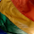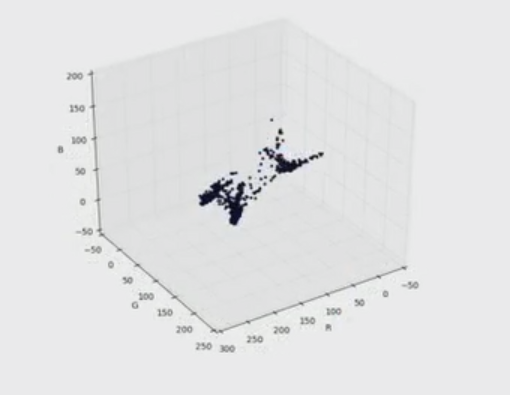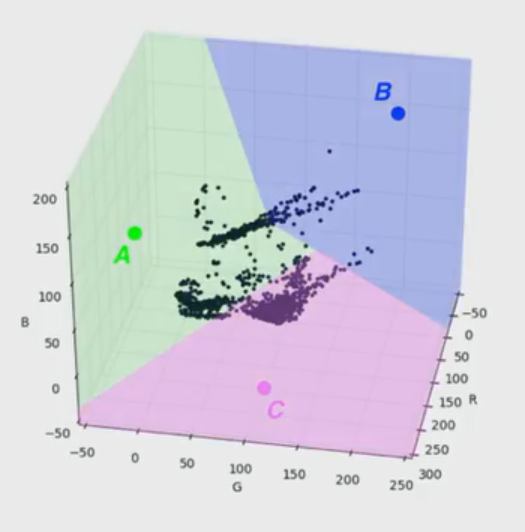#### 加载图片

``````import numpy as np
import matplotlib.pyplot as plt
import cv2

%matplotlib inline

## TODO: Check out the images directory to see other images you can work with
# And select one!

# Change color to RGB (from BGR)
image = cv2.cvtColor(image, cv2.COLOR_BGR2RGB)

plt.imshow(image)
``````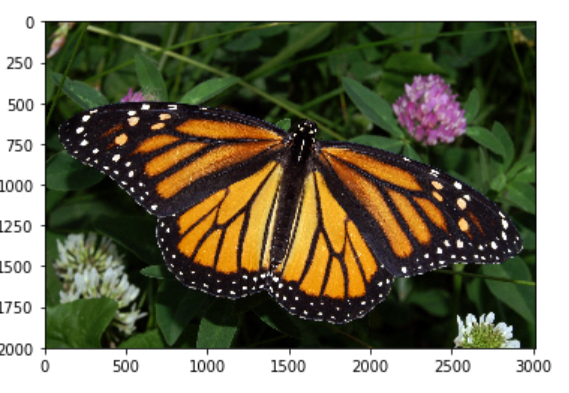#### K均值数据准备

``````# Reshape image into a 2D array of pixels and 3 color values (RGB)
pixel_vals = image.reshape((-1,3))

# Convert to float type
pixel_vals = np.float32(pixel_vals)
``````

#### 实现K均值聚类

``````## TODO: Visualize one segment, try to find which is the leaves, background, etc!
plt.imshow(labels_reshape==0, cmap='gray')
``````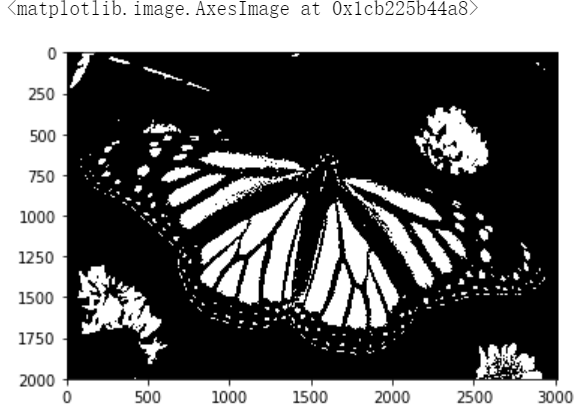``````# mask an image segment by cluster

cluster = 0                            # the first cluster

masked_image[labels_reshape == cluster] = [0, 255, 0]

``````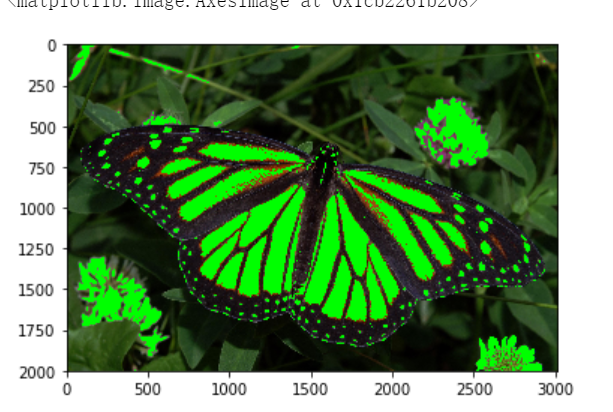``````# define stopping criteria
# you can change the number of max iterations for faster convergence!
criteria = (cv2.TERM_CRITERIA_EPS + cv2.TERM_CRITERIA_MAX_ITER, 100, 0.2)

## TODO: Select a value for k
# then perform k-means clustering
k = 3
retval, labels, centers = cv2.kmeans(pixel_vals, k, None, criteria, 10, cv2.KMEANS_RANDOM_CENTERS)

# convert data into 8-bit values
centers = np.uint8(centers)
segmented_data = centers[labels.flatten()]

# reshape data into the original image dimensions
segmented_image = segmented_data.reshape((image.shape))
labels_reshape = labels.reshape(image.shape, image.shape)

plt.imshow(segmented_image)
``````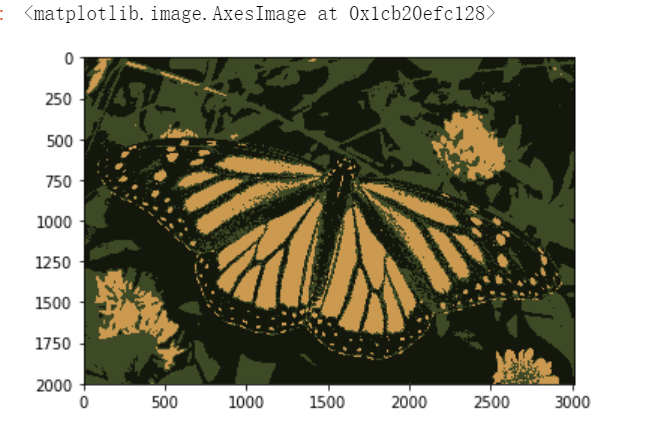K均值在图像分割中的作用十分明显，希望可以熟练应用~